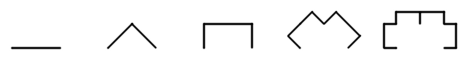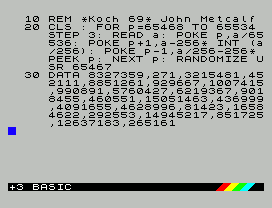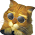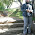## Thursday, 1 August 2013

### ZX Spectrum Koch (Lévy C) CurveA few years ago I submitted a couple of type-in programs (C-Curve and Curtains) to Your Sinclair and they featured in the penultimate issue (August 1993).

Encouraged by a shiny new YS badge I sent off a new batch of programs. Unfortunately it was too late. The September issue would be Your Sinclair's "Big Final Issue".

C-Curve is one of the simplest fractal curves. It starts with a straight line. To find the next iteration, each line is replaced by two lines at 90°:Here's a later 69 byte version of the program which plots the fractal in approximately 1.52 seconds! Assemble with Pasmo (`pasmo ccurve.asm ccurve.bin`), load the binary to address 65467 in your favourite emulator and run using `RANDOMIZE USR 65467` :-)

``` org 65467 ld de,49023 ; d = position on x axis ; e = position on y axis ld bc,3840 ; b = number of iterations ; c = initial direction RECURSE: djnz DOWN ld a,6 ; check direction and c ; c=0, left rrca ; c=2, up rrca ; c=4, right add a,a ; c=6, down dec a jr nc,XMOVE add a,e ; adjust y position +/-1 ld e,a ; calculate high byte of screen pos rrca scf rra rrca xor e and 88 xor e and 95 ld h,a sub h XMOVE: add a,d ; adjust x position +/-1 ld d,a ; calculate low byte of screen pos rlca rlca rlca xor e and 199 xor e rlca rlca ld l,a ld a,7 ; calculate bit position of pixel and d ld b,a inc b ld a,1 SHIFTBIT: rrca djnz SHIFTBIT xor (hl) ; plot ld (hl),a ret DOWN: inc c ; turn 45° clockwise call RECURSE inc b dec c ; turn 90° anti-clockwise dec c call RECURSE inc b inc c ; turn 45° clockwise ret ```

Finally here's a short type-in program to poke the code into a real Spectrum!1.Wow. Got to try that. Really 69 bytes? Amazing!

2.hey, if fractal building on the ZX Spectrum, why not to use ROM routines?;)
53 bytes! or less?

device zxspectrum128

org 65000;65467

begin
; call frac
; jr \$
frac:
ld de,49023 ; d = position on x axis
; e = position on y axis
ld bc,3840 ; b = number of iterations
; c = initial direction
RECURSE:
djnz DOWN

ld a,6 ; check direction
and c ; c=0, left
rrca ; c=2, up
rrca ; c=4, right
dec a
jr nc,XMOVE

ld e,a ; calculate high byte of screen pos

push bc
ld c,d
;;ld a,e
;A=y
;c=X
call 022B0h
inc a
ld b,a
xor a:scf
bwlp:rra:djnz bwlp
xor (hl):ld (hl),a
pop bc
xor a

; rrca
; scf
; rra
; rrca
; xor e
; and 88
; xor e
; and 95
; ld h,a
; sub h
;
XMOVE:

ld d,a ; calculate low byte of screen pos

; rlca
; rlca
; rlca
; xor e
; and 199
; xor e
; rlca
; rlca
; ld l,a
;
; ld a,7 ; calculate bit position of pixel
; and d
; ld b,a
ld b,0;inc b

; ld a,1
;SHIFTBIT:
; rrca
; djnz SHIFTBIT
;
; xor (hl) ; plot
; ld (hl),a
ret

DOWN:
inc c ; turn 45° clockwise
call RECURSE
inc b
dec c ; turn 90° anti-clockwise
dec c
call RECURSE
inc b
inc c ; turn 45° clockwise
ret

end
display /d,end-begin
savesna "frac.sna",begin

3.